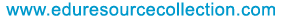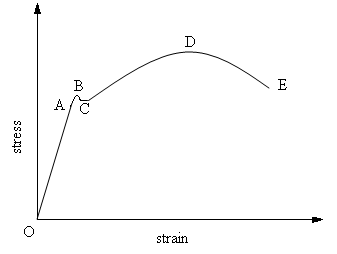@import url(http://www.google.com/cse/api/branding.css);Custom Search The content of the site is exclusively owned byHome | School Level | School Projects | Examinations | Civil | Food Processing | Seminars | Software Testing | Warehousing & Inventory | Networking

# Relationship between Stress and Strain

4. Relationship between Stress and Strain
Relationship between Stress and Strain are derived on the basis of the elastic behaviour of material bodies.

A standard mild steel specimen is subjected to a gradually increasing pull by Universal Testing Machine. The stress-strain curve obtained is as shown below.A -Elastic Limit
B - Upper Yield Stress
C - Lower Yield Stress
D -Ultimate Stress
E -Breaking Stress

4.1. Elasticity and Elastic Limit
Elasticity of a body is the property of the body by virtue of which the body regains its original size and shape when the deformation force is removed. Most materials are elastic in nature to a lesser or greater extend, even though perfectly elastic materials are very rare.

The maximum stress upto which a material can exhibit the property of elasticity is called the elastic limit. If the deformation forces applied causes the stress in the material to exceed the elastic limit, there will be a permanent set in it. That is the body will not regain its original shape and size even after the removal of the deforming force completely. There will be some residual strain left in it.

Yield stress
When a specimen is loaded beyond the elastic limit the stress increases and reach a point at which the material starts yielding this stress is called yield stress.

Ultimate stress
Ultimate load is defined as maximum load which can be placed prior to the breaking of the specimen. Stress corresponding to the ultimate load is known as ultimate stress.

Working stress
Working stress= Yield stress/Factor of safety.

4.2. Hooke’s Law
Hooke’s law states that stress is proportional to strain upto elastic limit. If I is the stress induced in a material and e the corresponding strain, then according to Hooke’s law,
p / e = E, a constant.

This constant E is called the modulus of elasticity or Young’s Modulus, (named after the English scientist Thomas Young).
It has later been established that Hooke’s law is valid only upto a stress called the limit of proportionality which is slightly less than the elastic limit.

4.3. Elastic Constants
Elastic constants are used to express the relationship between stresses and strains. Hooke’s law , is stress/strain = a constant, within a certain limit. This means that any stress/corresponding strain = a constant, within certain limit. It follows that there can be three different types of such constants. (which we may call the elastic constants or elastic modulae) corresponding to three distinct types of stresses and strains. These are given below.

(i)Modulus of Elasticity or Young’s Modulus (E)
Modulus of Elasticity is the ratio of direct stress to corresponding linear strain within elastic limit. If p is any direct stress below the elastic limit and e the corresponding linear strain, then E = p / e.

(ii)Modulus of Rigidity or Shear Modulus (G)
Modulus of Rigidity is the ratio of shear stress to shear strain within elastic limit. It is denoted by N,C or G. if q is the shear stress within elastic limit and f the corresponding shear strain, then G = q / f.

(iii) Bulk Modulus (K)
Bulk Modulus is the ratio of volumetric stress to volumetric strain within the elastic limit. If pv is the volumetric stress within elastic limit and ev the corresponding volumetric strain, we have K = pv / ev.